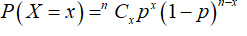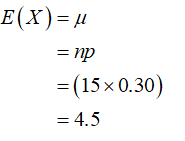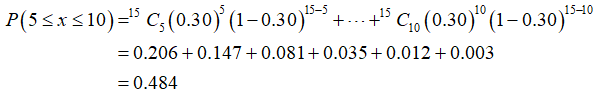# Suppose that 30% of all students who have to buy a text for a particular course want a new copy (the successes!), whereas the other 70% want a used copy. Consider randomly selecting 15 purchasers. (c)The bookstore has 10 new copies and 10 used copies in stock. If 15 people come in one by one to purchase this text, what is the probability that all 15 will get the type of book they want from current stock? [Hint: Let X = the number who want a new copy. For what values of X will all 15 get what they want?] (Round your answer to three decimal places.) (d)Suppose that new copies cost \$130 and used copies cost \$80. Assume the bookstore currently has 50 new copies and 50 used copies. What is the expected value of total revenue from the sale of the next 15 copies purchased? [Hint: Let h(X) = the revenue when X of the 15 purchasers want new copies. Express this as a linear function.]

Question
38 views
Suppose that 30% of all students who have to buy a text for a particular course want a new copy (the successes!), whereas the other 70% want a used copy. Consider randomly selecting 15 purchasers.

(c)
The bookstore has 10 new copies and 10 used copies in stock. If 15 people come in one by one to purchase this text, what is the probability that all 15 will get the type of book they want from current stock? [Hint: Let X = the number who want a new copy. For what values of X will all 15 get what they want?] (Round your answer to three decimal places.)

(d)
Suppose that new copies cost \$130 and used copies cost \$80. Assume the bookstore currently has 50 new copies and 50 used copies. What is the expected value of total revenue from the sale of the next 15 copies purchased? [Hint: Let h(X) = the revenue when X of the 15 purchasers want new copies. Express this as a linear function.]
check_circle

Step 1

c). From the provided information,

The sample size (n) = 15

Probability of successes (p) = 0.30

The process will follow binomial distribution with probability distribution function as follow:The mean value can be obtained as:The required probability that all 15 will get the type of book they want from current stock (probability at most 10 and at least 5 people want new book) can be obtained as:Thus, the required probability is 0.484.

...

### Want to see the full answer?

See Solution

#### Want to see this answer and more?

Solutions are written by subject experts who are available 24/7. Questions are typically answered within 1 hour.*

See Solution
*Response times may vary by subject and question.
Tagged in